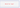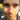### Lanky Dan Blog# Which for loop?

January 15, 2017

javajava 8

Java 8 has introduced another type of `for` loop. This gives us the third way to use them. You would think that releasing another way to use the loop would suggest that it must be better than the others. But that is not the case, each one has subtle differences meaning you should have a little think before you decide which one to use.

Types of for loops:

• Normal `for` loop
• `for-each` loop
• Java 8 `for-each` loop

## Normal for loop

Now I’m just going to come straight out and say this. These loops look ugly and I try to stay away from them as much as possible. Anyway now I have said that lets get back to explaining what they are. This type of loop uses a counter to iterate through the elements of a list or array. The easiest way to explain it is through an example.

``````List<Integer> list = Arrays.asList(1,2,3,4,5,6);
for(int index=0; index<list.size(); index++) {
System.out.println("I have read the number: " + list.get(index));
}

int[] array = new int[]{ 1,2,3,4,5,6};
for(int index=0; index<array.length; index++) {
System.out.println("I have read the number: " + array[index]);
}``````

The above shows how to iterate through both a list and an array. It does so by increasing the counters value `index` and retrieving the element at that position using `list.get(index)` or `array[index]`. After it has the element it can do whatever it wants with it. The advantage of using this type of loop is that you have more control over how you read the elements than you do with the other types of loops. If you wanted you could retrieve the element at position `index-1` very easily, just remember to handle the scenario of `index = 0` or your going to have a `IndexOutOfBoundsException` on your hands. This advantage of control is also its downside as you need to manually retrieve the elements you need which makes the code look cluttered. The ugliness of this loop is not found in the other types of loops.

## The for-each loop

The name of this loop pretty much sums up what it does. For each element in the collection or array do something with it. The is no need to increment through the list using a counter as that is all done behind the scenes. Simply give the object you want to retrieve and type and name and you are good to go.

``````List<Integer> list = Arrays.asList(1,2,3,4,5,6);
for(int number : list) {
System.out.println("I have read the number:" + number);
}

int[] array = new int[]{ 1,2,3,4,5,6};
for(int number : array) {
System.out.println("I have read the number: " + number);
}

Set<Integer> set = new HashSet<>(Arrays.asList(1,2,3,4,5,6));
for(int number : set) {
System.out.println("I have read the number: " + number);
}``````

Look how simple and pretty they are, so much nicer than those ugly loops above. Anyway, the loops will go through the elements and during each iteration provide the defined variable a value from the collection or array. The order in which the values are retrieved follow the same order that the elements have in the collection or array. Another advantage of the `for-each` loop is that it can iterate through the elements of a `Set` as it does not have its own `get()` method.

## The Java 8 for-each loop

This is the new `for` loop that has been added with the release of Java 8. It has all the characteristics of the normal `for-each` loop but uses Lambda expressions to define what it does with each element it iterates over from the collection, although this form cannot be used on an array. The example below is pretty self explanatory.

``````List<Integer> list = Arrays.asList(1,2,3,4,5,6);
list.forEach(number -> System.out.println("I have read the number:" + number));``````

Using this form of `for` of loop can be used instead of the normal `for-each` loop as functionally they are the same, but the syntax is different. This form looks nicer for simple lambda expressions and can allow a loop to be defined in a single line if the expression is small enough. Although for loops with more statements inside them it is the users preference in which `for-each` loop they use as the benefit of decreasing the amount of code written is no longer there`

So which loop should you use? If you need total control over the elements you read or need to add and remove elements from the list, then a normal `for` loop is the way to go. If you simply need to perform some statements on elements of the list and don’t need to add or remove elements, then both the normal `for-each` and Java 8 `for-each` loops will suffice. Determining which `for-each` loop to use is up to you, personally for singular or smaller statements I would use the Java 8 `for-each` loop but for loops with longer statements inside then the good old normal `for-each` will be my loop of choice.Written by Dan Newton.